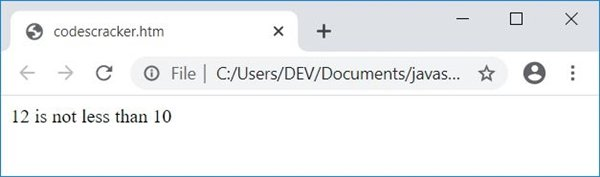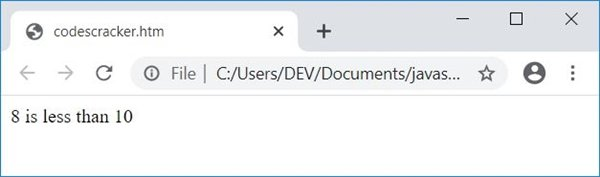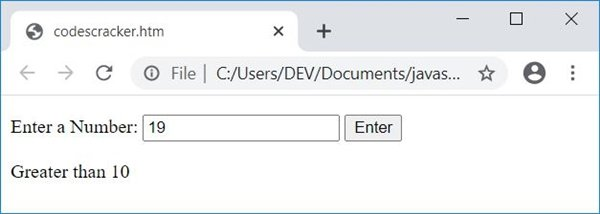# JavaScript Programming Examples

JavaScript is currently one of the most in-demand programming languages worldwide. The most widely used and well-known programming language in the world is JavaScript.

As a result, we are offering numerous JavaScript programs for practice and learning. The output for each and every JavaScript program is provided.

The number of programs that can be made with JavaScript is excessive. A series of posts on JavaScript programs has been created because it is impossible to attach all the programs to a single article.

## JavaScript Programs for Beginners

We've provided the simplest JavaScript code to do the task in each JavaScript program. So that even a novice JavaScript learner can grasp the subject. Later on, user input is received, and calculations are performed based on that input.

For example, if the user wants to create a code to add two numbers in JavaScript, he or she can use the following JavaScript code:

```var a = 3;
var b = 7;
var sum = a + b;```

In the above JavaScript two lines of code, the first line declares three variables, a, b, and sum. Values 3 and 7 are already initialized for the first two variables. Whereas in the second line, the value a+b, 3+7, or 10 gets initialized to sum.

#### Top JavaScript Programs

Here is a list of some popular programs in JavaScript:

## JavaScript Program Examples with Output

Every JavaScript program written in this series is well tested with its output. For example, let's have a look at the following JavaScript program to check whether the number is less than 10 or not:

```var num=12;
if(num<10)
document.write(num + " is less than 10");
else
document.write(num + " is not less than 10");```

The provided code creates a "num" variable and gives it the value of 12. It then checks to see if the value of the variable "num" is less than 10 or not using an if-else statement.

The program will run the statement inside the "if" block, which in this case is to write "num is less than 10" to the document using the document.write() method, if the value of "num" is less than 10.

The program will run the statement contained in the "else" block if the value of "num" is greater than 10, in which case it will use the "document.write()" method to write "num is not less than 10" to the document.

Due to the fact that "num" in this example has a value of 12, which is greater than 10, the "else" block will be executed, and the program's output will read "12 is not less than 10."

Note: I'm using the "document.write()" method only for the example purpose, but you can choose the console.log() method to see the output on the browser console.

This is the simplest JavaScript code, which shows the demo of how the simplest JavaScript code is created in each and every article for beginner learners. And here is the same JavaScript program written along with HTML:

```<!doctype html>
<html>
<script>
var num=12;
if(num<10)
document.write(num + " is less than 10");
else
document.write(num + " is not less than 10");
</script>
<body>

</body>
</html>```

Save the above code in a file with .html extension, say codescracker.html. Now open this file in a web browser. Here is the output you will see:Now change the value of num to 8 and save the code in the same file. Therefore, after refreshing the web browser, here is the output you will see this time:Note: The document.write() method writes data inside its braces () to an HTML output.

Finally, this is the actual program that receives input from the user, and then JavaScript code gets into action based on user input:

```<!doctype html>
<html>
<script>
function jsFun()
{
var val, elem;
val = parseInt(document.getElementById("num").value);
if(val)
{
if(val<10)
document.getElementById("paraTwo").innerHTML = "Less than 10";
else
document.getElementById("paraTwo").innerHTML = "Greater than 10";
}
}
</script>
<body>

<p id="paraOne">Enter a Number: <input id="num">
<button onclick="jsFun()">Enter</button></p>
<p id="paraTwo"></p>

</body>
</html>```

Here is its sample output:Now enter a number, say 19, and click on the Enter button to see the following output:#### Live Output of the Previous JavaScript Program

Here is the live output that the preceding example produced:

Enter a Number:

When the user clicks on the button Enter, a function named jsFun() gets called. And all the statements of this function get executed. That is, two variables, val and elem, get declared, and the statement:

`val = parseInt(document.getElementById("num").value);`

states that an int (integer) value of an HTML element whose id is num gets initialized to val variable. Now the following code:
`if(val)`
checks whether val has some value or is empty. This code is created to check whether the user enters some data, then clicks on the Enter button, or clicks on the Enter button without providing the input.

Now if the user enters the number, say 19, then val=19. Now, using if, we've checked whether the value of val (19) is less than 10 or not. Because the condition, val<10 or 19<10 evaluates to false, program flow does not go inside the if's body, but rather to its else's part, and the statement:

`document.getElementById("paraTwo").innerHTML = "Greater than 10";`

gets executed, which states that the value of an HTML element whose id is paraTwo gets printed as Greater than 10.

JavaScript Online Test

« JavaScript Tutorial Next Program »